Chapter 17. Kinetics

# End-of-Chapter Material

Jessie A. Key

1. What factors affect the rate of a reaction?
2. How does a decrease in temperature affect the reaction rate? Explain the outcome by describing changes that occur at the molecular level.
3. For which of the following two reactions would you expect the orientation of the molecules to be more important?
1. AB + C → AC + B
2. D + E → F
4. Determine the relative rates of disappearance of reactants and formation of products for the following reactions:
1. N2(g) + 3H2(g) → 2NH3(g)
2. 2A + 3B → 4C
3. 2N2O5 → 4 NO2 + O2
5. Methanol reacts with hydrochloric acid to produce methyl chloride and water by the following reaction:

CH3OH(aq) + HCl(aq) → CH3Cl(aq) + H2O(ℓ)
The following data were obtained for this reaction at a particular temperature:

Time (min) [CH3OH] (M)
0.0 1.95
60.0 1.50
120.0 1.24
180.0 1.04
240.0 0.85
300.0 0.70
1. Calculate the average reaction rate between 0 and 60 minutes and 180 and 240 minutes. Which is faster? Explain using your knowledge of factors that can affect rate.
2. Determine the instantaneous rate at 200 minutes.
6. For the following reaction: 3 E + 2 F → 2 G, the rate law is Rate = k[F]2.
1. How does the rate change if [E] is doubled?
2. How does the rate change is [F] is doubled?
3. What is the overall reaction order?
4. What are the units for the rate constant for this reaction?
7. The rate of oxidation of bromide ions by bromate in an acidic aqueous solution is

6 H+ + BrO3 + 5Br → 3Br2 + 3H2O

and is found to follow the rate law

Rate = k[Br][BrO3][H+]2

What happens to the rate if, in separate experiments:

1. [BrO3] is doubled?
2. the pH is increased by one unit?
3. the solution is diluted to twice its volume, with the pH kept constant by use of a buffer?
8. The following data was obtained using the initial rate method:

A + B → C + D

Experiment [A] (M) [B] (M) Initial Rate (M/s)
1 2.0 × 10−3 2.0 × 10−3 1.45 × 10−4
2 4.0 × 10−3 2.0 × 10−3 2.90 × 10−4
3 2.0 × 10−3 4.0 × 10−3 2.90 × 10−4
1. Determine the rate law for this equation.
2. Calculate the rate constant, including units.
3. Determine the rate when [A] = 0.250 M and [B] = 0.100 M.
9. A study of the gas-phase reduction of nitric oxide by hydrogen

2NO + 2H2 → N2 + 2H2O

yielded the following initial-rate data (all pressures in torr):

Experiment P(NO) P(H2) Initial Rate (torr s−1)
1 359 300 1.50
2 300 300 1.03
3 152 300 0.25
4 300 289 1.00
5 300 205 0.71
6 200 147 0.51

Find the order of the reaction with respect to each component.

10. What should be plotted on the x– and y-axis to obtain a straight line for:
1. a first-order reaction?
2. a zero-order reaction?
11. What does the slope represent for:
1. a second-order reaction kinetic plot?
2. a first-order reaction kinetic plot?
12. Common sugar, sucrose C12H22O11, reacts in dilute acidic solutions to produce glucose and fructose, both having the molecular formula C6H12O6.

C12H22O11(aq) + H2O(ℓ) → 2C6H12O6(aq)

During an experiment, the following data were obtained:

Time (hours) [Sucrose] (mM)
0.00 316
0.65 274
1.33 238
2.33 190
3.50 146
1. What is the order of the reaction?
2. Determine the rate law and the rate constant.
3. Calculate the expected concentration of sucrose after 100 min.
13. The half-life of a first-order reaction was found to be 10 min at a certain temperature. What is its rate constant in reciprocal seconds?
14. The mass-241 isotope of americium, widely used as an ionizing source in smoke detectors, has a half-life of 432 years.
1. What fraction of the Am241 in a smoke detector will have decayed after 50 years?
2. How long will it take for the activity to decline to 80% of its initial value?
3. What would be the “seventh-life” of Am241?
15. A widely used “rule of thumb” for the temperature dependence of a reaction rate is that a 10°C rise in the temperature approximately doubles the rate. (This is obviously not generally true, especially when a strong covalent bond must be broken.) For a reaction that shows this behaviour, what would the activation energy be?
16. The temperature dependence of the rate constant, k, for a reaction was found to be:
Temperature (K) k (M−1s−1)
500 0.025
600 0.300
700 1.500
800 7.000
900 28.000

Calculate the activation energy (Ea) and A.

17. State the molecularity and rate law for each of the following elementary steps:
1. Cl(g) + CCl3(g) → CCl4(ℓ)
2. Br2 → 2Br
3. 2A → B
18. A reaction is believed to occur by the following two elementary steps:

2NO2 → NO3 + NO

NO3 → NO + O2

With this in mind:

1. Write the balanced overall equation of this reaction.
2. Identify any intermediates in the mechanism.
3. If the first step is much slower than the second, what would you expect the rate law for the overall reaction to be?
19. Use the following potential energy diagram to answer questions 19a to 19c:
1. How many steps are in this mechanism?
2. How many intermediates are there in this reaction?
3. What is the slowest step?
20. What is a catalyst? Explain how a catalyst affects reaction rates.
21. Many solid catalysts are commercially sold as fine powders or small spherical beads. Explain why this might be beneficial.

1. temperature, reactant concentrations, physical state (surface area of solids), presence of a catalyst
1. AB + C → AC + B
1. average reaction rate 0–60 minutes: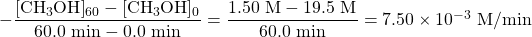average reaction rate 180–240 minutes: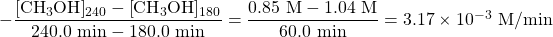Therefore the average reaction rate between 0–60 minutes is faster. This is because, as the reaction proceeds, the reactant gets consumed to make product, lowering the concentration of reactants.

2.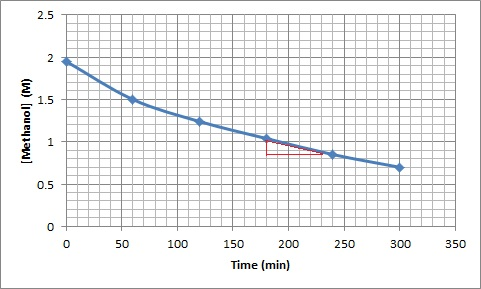Instantaneous rate at 200 minutes: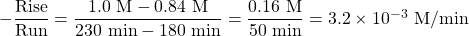1. Since the rate is first-order in bromate, doubling its concentration will double the reaction rate.
2. Increasing the pH by one unit will decrease the [H+] by a factor of 10. Since the reaction is second-order in [H+], this will decrease the rate by a factor of 100.
3. Dilution reduces the concentrations of both Br2 and BrO3 to half their original values. Doing this to each concentration alone would reduce the rate by a factor of 2, so reducing both concentrations will reduce the rate by a factor of 4, to (½) × (½) = ¼ of its initial value.
1. Experiments 2 and 3: Reduction of the initial partial pressure of NO by a factor of about 2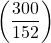results in a reduction of the initial rate by a factor of about 4, so the reaction is second-order in nitric oxide.
2. Experiments 4 and 6: Reducing the initial partial pressure of hydrogen by a factor of approximately 2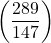causes a similar reduction in the initial rate, so the reaction is first-order in hydrogen.
3. The rate law is: rate = k[NO]2[H2].
1. k
2. k
1. From the above equation,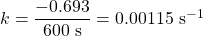1. We will centre our ten-degree interval at 300 K. Substituting into the above expression yields: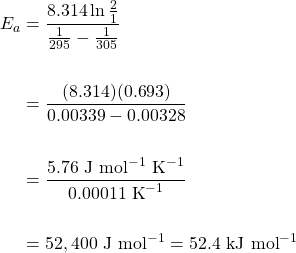1. bimolecular, rate = k[Cl] [CCl3]
2. unimolecular, rate = k[Br2]
3. bimolecular 2A, rate = k[A]2
1. 3 steps
2. 2 intermediates
3. The second step is the slowest step
1. Finely divided powders and spherical beads have larger surface areas than big solid chunks, allowing for more sites for reactions to occur, which further speeds up the reaction.

1. Question and solution from Chem1 Virtual Textbook, Stephen Lower/CC-BY-SA-3.0
2. Question and solution from Chem1 Virtual Textbook, Stephen Lower/CC-BY-SA-3.0
3. Question and solution from Chem1 Virtual Textbook, Stephen Lower/CC-BY-SA-3.0
4. Question and solution from Chem1 Virtual Textbook, Stephen Lower/CC-BY-SA-3.0
5. Question and solution from Chem1 Virtual Textbook, Stephen Lower/CC-BY-SA-3.0